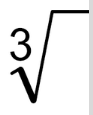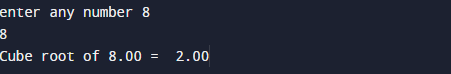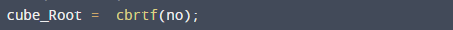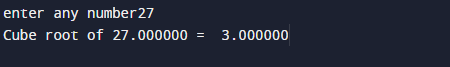C Program to Find Cube Root of a Given Number

In this tutorial you will learn about the C Program to Find Cube Root of a Given Number and its application with practical example.

In this tutorial, we will learn to create a C program that will find Cube Root of given number  using C programming.

Prerequisites

Before starting with this tutorial we assume that you are best aware of the following C programming topics:

• C Operators.
• Basic input/output.
• In-built functions.
• mathematical functions.

What Is cube root?

Cube root , is a factor that we multiply by itself three times to get that number (2*2*2=8).C Program to Find Cube Root of a Given Number.

Here in this program we are going find cube root of a given number first of all we take a value from user and pass this value to in-built function to find cube root of a number.

OutputYou can  also use following function like  cbrt() with double and crbtl() with long double  data type.

Function cbrtf() takes only single  parameter(no) and returns the cube root.which we hold in cube_root variable.with the help of cube_root  variable we can print the cube of given number of userAlgorithm

Algorithm for  this program is:−

START
1: →Take a variable no.
2 :→Take value from user and assign to no.
3 :→ Pass no variable to cube_root function (cbrtf(no)).
4 :→ This function returns value to cube_root variable.
5 :→ Print the value of cube_root.
STOP
Explanation of Cube root, cube root of a number is a value ,when multiplied by itself 3 times .

For example.
The cube root of number 27, denoted as 3√27, is 3,
because when we multiply 3 by itself thrice times we get  3 x 3 x 3 = 27 = 33.

In this tutorial we have learn about the C Program to Find Cube Root of a Given Number and its application with practical example. I hope you will like this tutorial.